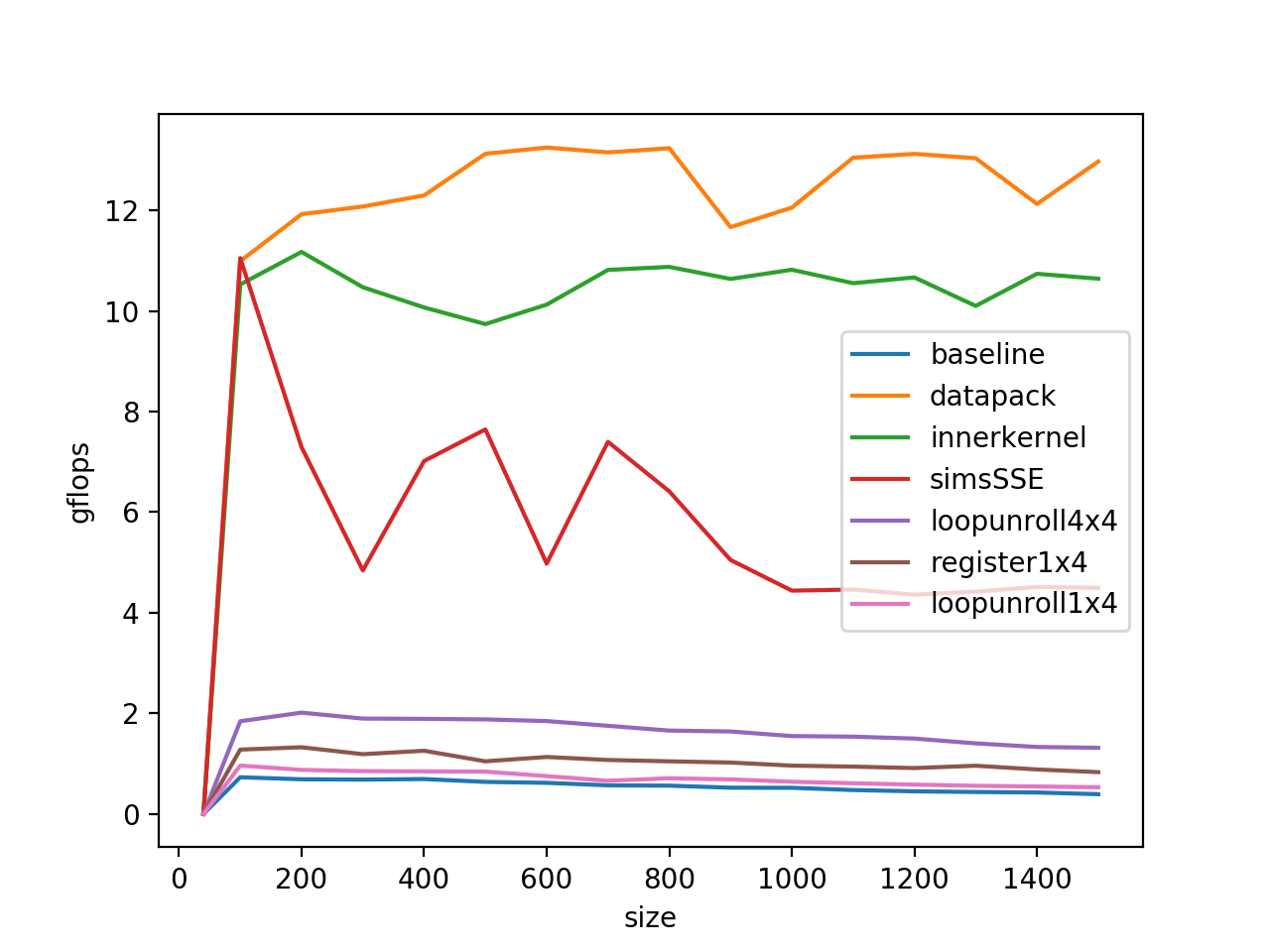how-to-optimiza-gemm 是大家参考得比较多的gemm优化tutorial，本文是在我的MacBook Pro 2019上进行的实践，处理器型号是i5-8257U.

### 1.浮点峰值计算

❯ sysctl machdep.cpu.brand_string
machdep.cpu.brand_string: Intel(R) Core(TM) i5-8257U CPU @ 1.40GHzstatic void cpuid_x86_exec(struct cpuid_t *cpuid) {
asm volatile("pushq %%rbx\n"
"cpuid\n"
"movl %%ebx, %1\n"
"popq %%rbx\n"
: "=a"(cpuid->eax), "=r"(cpuid->ebx), "=c"(cpuid->ecx), "=d"(cpuid->edx)
: "a"(cpuid->ieax), "c"(cpuid->iecx)
: "cc");
}

struct cpuid_t cpuid;

feat = 0;

cpuid.ieax = 0x1;
cpuid.iecx = 0x0;
cpuid_x86_exec(&cpuid);

if (BIT_TEST(cpuid.edx, 25)) {
SET_FEAT(_CPUID_X86_SSE_);
}
if (BIT_TEST(cpuid.ecx, 28)) {
SET_FEAT(_CPUID_X86_AVX_);
}
if (BIT_TEST(cpuid.ecx, 12)) {
SET_FEAT(_CPUID_X86_FMA_);
}

cpuid.ieax = 0x7;
cpuid.iecx = 0x0;
cpuid_x86_exec(&cpuid);

if (BIT_TEST(cpuid.ebx, 16)) {
SET_FEAT(_CPUID_X86_AVX512F_);
}
if (BIT_TEST(cpuid.ecx, 11)) {
SET_FEAT(_CPUID_X86_AVX512_VNNI_);
}

H，M,U，表示功耗，字母越小，功耗越大，性能越好。所以后缀：H>M>U。比如：i5-5350H>i7-4610m,i5-4330m>i7-4558U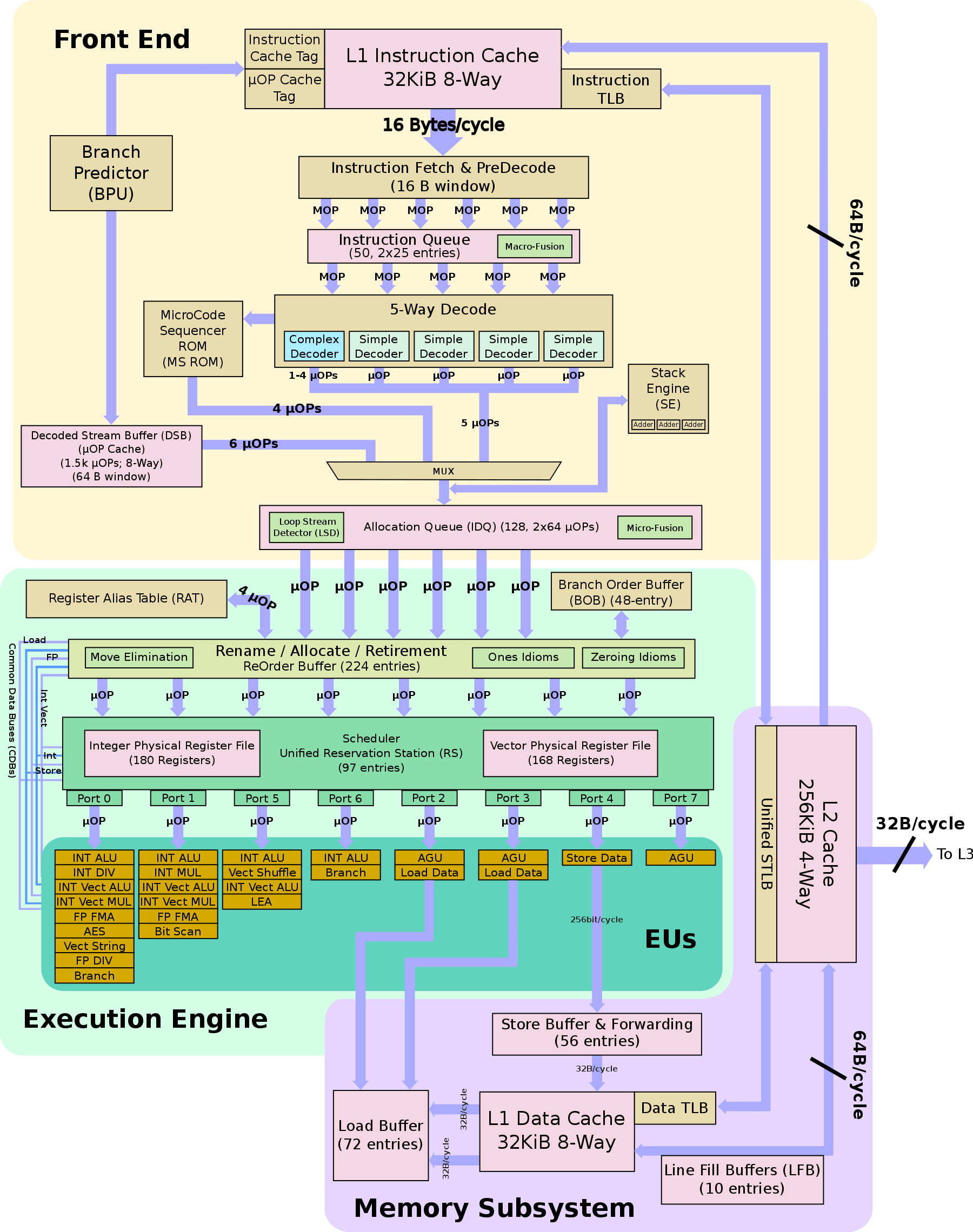PORT0和PORT1可以同时执行向量乘、向量加和FMA指令，通过查datasheet可以知道：

$$2(256/32)2*1.4=44.8 \quad GFLOPS$$

Mac上需要使用到 Turbo Boost Switcher Pro这个软件来关闭睿频，不过我的mbp2019日常开启睿频，风扇疯狂起飞XDD

$$2(256/32)2*3.9=124.8 \quad GFLOPS$$

gemm-optimizer/cmake-build-debug/tools/calc-cpu-flops 4

❯ tree
.
├── CMakeLists.txt
├── calc-cpu-flops.c
├── cpufp_kernel_x86.h
├── cpufp_kernel_x86_avx.s
├── cpufp_kernel_x86_avx512_vnni.s
├── cpufp_kernel_x86_avx512f.s
├── cpufp_kernel_x86_fma.s
├── cpufp_kernel_x86_sse.s
├── smtl.c
└── smtl.h

_cpufp_kernel_x86_fma_fp32:
mov $0x40000000, %rax vxorps %ymm0, %ymm0, %ymm0 vxorps %ymm1, %ymm1, %ymm1 vxorps %ymm2, %ymm2, %ymm2 vxorps %ymm3, %ymm3, %ymm3 vxorps %ymm4, %ymm4, %ymm4 vxorps %ymm5, %ymm5, %ymm5 vxorps %ymm6, %ymm6, %ymm6 vxorps %ymm7, %ymm7, %ymm7 vxorps %ymm8, %ymm8, %ymm8 vxorps %ymm9, %ymm9, %ymm9 ._cpufp.x86.fma.fp32.L1: vfmadd231ps %ymm0, %ymm0, %ymm0 vfmadd231ps %ymm1, %ymm1, %ymm1 vfmadd231ps %ymm2, %ymm2, %ymm2 vfmadd231ps %ymm3, %ymm3, %ymm3 vfmadd231ps %ymm4, %ymm4, %ymm4 vfmadd231ps %ymm5, %ymm5, %ymm5 vfmadd231ps %ymm6, %ymm6, %ymm6 vfmadd231ps %ymm7, %ymm7, %ymm7 vfmadd231ps %ymm8, %ymm8, %ymm8 vfmadd231ps %ymm9, %ymm9, %ymm9 sub$0x1, %rax
jne ._cpufp.x86.fma.fp32.L1
ret

rax存储的是循环的次数，循环初始化的时候执行的一系列异或指令是用来快速将寄存器的内容置零，接着循环内会执行十次乘累加运算，一共执行40000000次，之前分析得，对于32位的浮点数， 一次乘累加运算可以操作8个数，一共是 40000000 * 8 * 10 * 2（乘法和加法）运算，共这么大的浮点运算量：

#ifdef _FMA_
#define FMA_FP32_COMP (0x40000000L * 160)
#define FMA_FP64_COMP (0x40000000L * 80)

perf = FMA_FP64_COMP * num_threads / time_used * 1e-9;

Thread(s): 1
binding to core 0
fma fp32 perf: 40.9189 gflops.
fma fp64 perf: 22.0921 gflops.
binding to core 0
avx fp32 perf: 21.9944 gflops.
avx fp64 perf: 11.0741 gflops.
binding to core 0
sse fp32 perf: 10.8526 gfops.
sse fp64 perf: 5.4786 gflops.

Thread(s): 1
binding to core 0
fma fp32 perf: 117.0210 gflops.
fma fp64 perf: 58.4361 gflops.
binding to core 0
avx fp32 perf: 56.1949 gflops.
avx fp64 perf: 28.9646 gflops.
binding to core 0
sse fp32 perf: 29.1122 gflops.
sse fp64 perf: 14.6471 gflops.

### 2.baseline

void AddDot( int k, double *x, int incx,  double *y, double *gamma )
{
/* compute gamma := x' * y + gamma with vectors x and y of length n.

Here x starts at location x with increment (stride) incx and y starts at location y and has (implicit) stride of 1.
*/

int p;

for ( p=0; p<k; p++ ){
*gamma += X( p ) * y[ p ];
}
}

/* Routine for computing C = A * B + C */

void mMultBaseLine( int m, int n, int k, double *a, int lda,
double *b, int ldb,
double *c, int ldc )
{
int i, j, p;

for ( i=0; i<m; i++ ){        /* Loop over the rows of C */
for ( j=0; j<n; j++ ){        /* Loop over the columns of C */
AddDot( k, &A( i,0 ), lda, &B( 0,j ), &C( i,j ) );
}
}
}


#define PFIRST 100
#define PLAST 1500
#define PINC 100

100 7.299270e-01 0.000000e+00
200 6.892096e-01 0.000000e+00
300 6.828183e-01 0.000000e+00
400 6.939813e-01 0.000000e+00
500 6.374153e-01 0.000000e+00
600 6.188340e-01 0.000000e+00
700 5.687877e-01 0.000000e+00
800 5.626816e-01 0.000000e+00
900 5.218054e-01 0.000000e+00
1000 5.200376e-01 0.000000e+00
1100 4.751246e-01 0.000000e+00
1200 4.499168e-01 0.000000e+00
1300 4.366850e-01 0.000000e+00
1400 4.270737e-01 0.000000e+00
1500 3.929416e-01 0.000000e+00 

tools/plot下我提供了一个py程序，用来绘制benckmark的：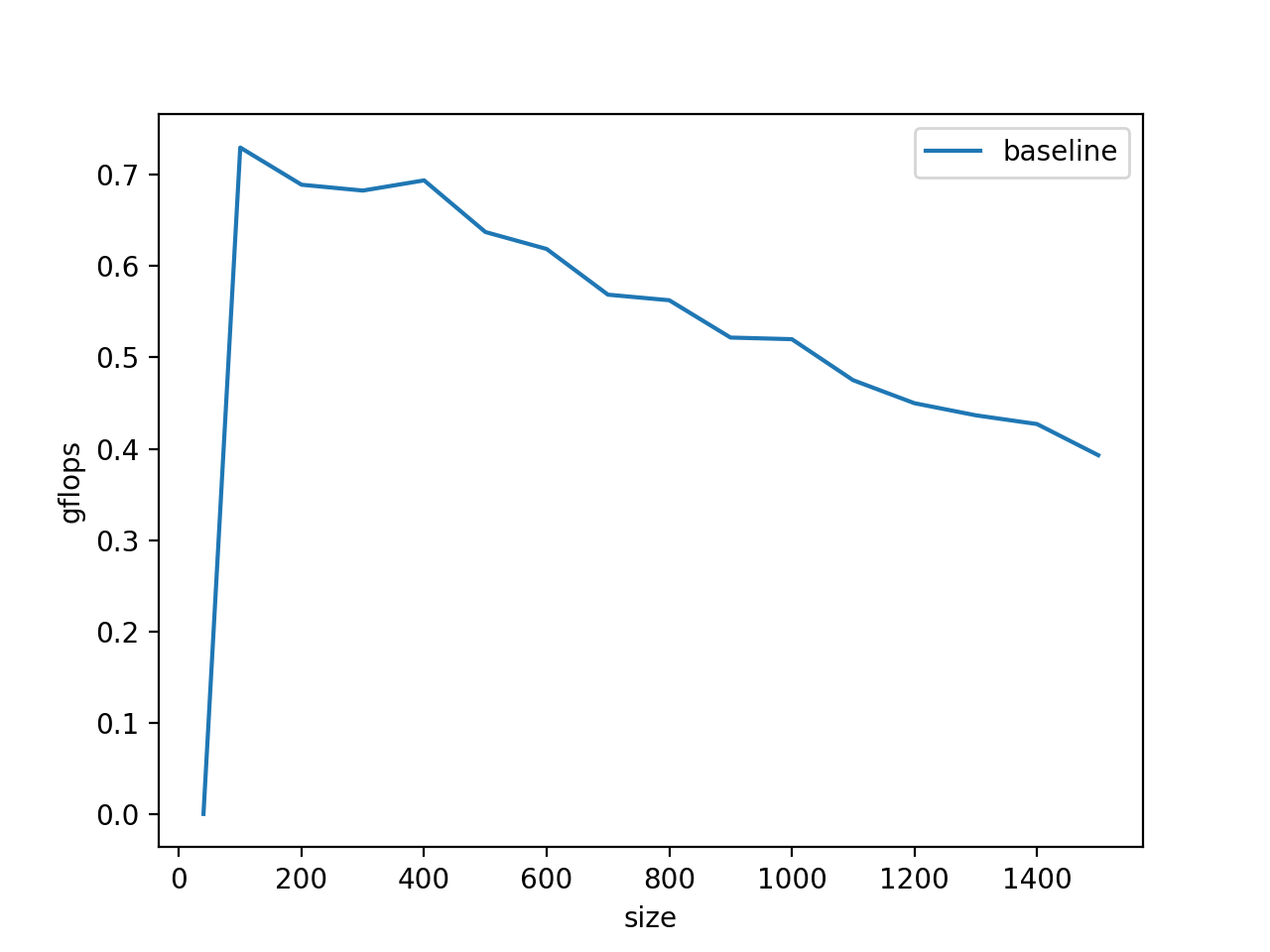gflops只有0.6，并且显而易见在gemm的尺寸变大的时候，gflop有下降趋势，这是因为当运算过程中的矩阵A、B的大小超过了L2 Cache的大小(256Kb)的时候，频繁访问A、B就会导致Cache要重复的访问DDR，变成访存的瓶颈，这部分可以通过矩阵分块计算来解决。

### 3.循环展开和合并

void MY_MMult( int m, int n, int k, double *a, int lda,
double *b, int ldb,
double *c, int ldc )
{
int i, j;

for ( j=0; j<n; j+=4 ){        /* Loop over the columns of C, unrolled by 4 */
for ( i=0; i<m; i+=1 ){        /* Loop over the rows of C */
/* Update C( i,j ), C( i,j+1 ), C( i,j+2 ), and C( i,j+3 ) in
one routine (four inner products) */
AddDot( k, &A( 0, 0 ), lda, &B( 0, 0 ), &C( 0, 0 ) );
AddDot( k, &A( 0, 0 ), lda, &B( 0, 1 ), &C( 0, 1 ) );
AddDot( k, &A( 0, 0 ), lda, &B( 0, 2 ), &C( 0, 2 ) );
AddDot( k, &A( 0, 0 ), lda, &B( 0, 3 ), &C( 0, 3 ) );
}
}
}


void MY_MMult( int m, int n, int k, double *a, int lda,
double *b, int ldb,
double *c, int ldc )
{
int i, j;

for ( j=0; j<n; j+=4 ){        /* Loop over the columns of C, unrolled by 4 */
for ( i=0; i<m; i+=1 ){        /* Loop over the rows of C */
int p;

//  AddDot( k, &A( 0, 0 ), lda, &B( 0, 0 ), &C( 0, 0 ) );
for ( p=0; p<k; p++ ){
C( 0, 0 ) += A( 0, p ) * B( p, 0 );
}

//  AddDot( k, &A( 0, 0 ), lda, &B( 0, 1 ), &C( 0, 1 ) );
for ( p=0; p<k; p++ ){
C( 0, 1 ) += A( 0, p ) * B( p, 1 );
}

//  AddDot( k, &A( 0, 0 ), lda, &B( 0, 2 ), &C( 0, 2 ) );
for ( p=0; p<k; p++ ){
C( 0, 2 ) += A( 0, p ) * B( p, 2 );
}

//  AddDot( k, &A( 0, 0 ), lda, &B( 0, 3 ), &C( 0, 3 ) );
for ( p=0; p<k; p++ ){
C( 0, 3 ) += A( 0, p ) * B( p, 3 );
}
}
}
}

for ( p=0; p<k; p++ ){
C( 0, 0 ) += A( 0, p ) * B( p, 0 );
C( 0, 1 ) += A( 0, p ) * B( p, 1 );
C( 0, 2 ) += A( 0, p ) * B( p, 2 );
C( 0, 3 ) += A( 0, p ) * B( p, 3 );
}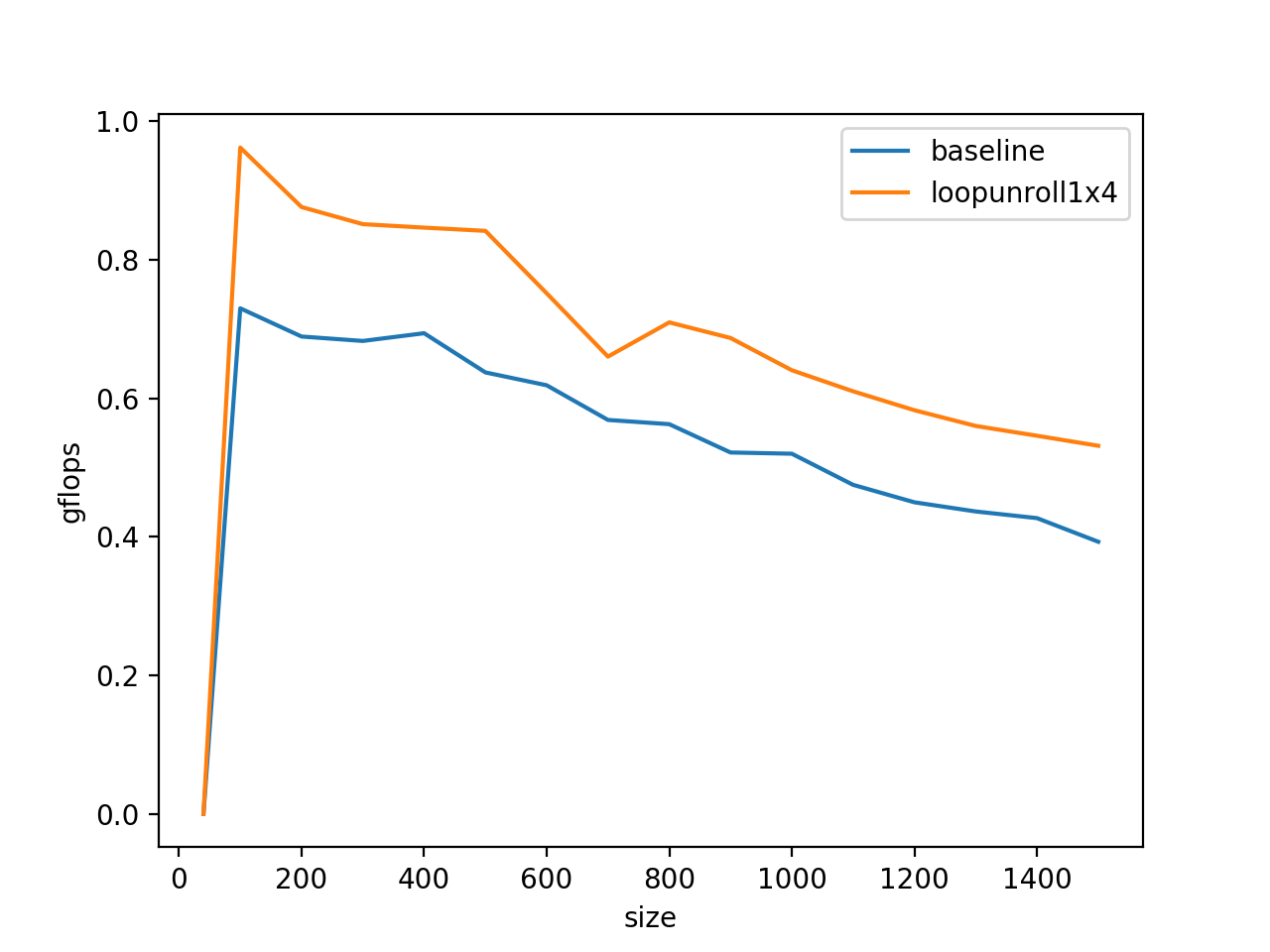### 4.访存方式

for ( p=0; p<k; p++ ){
C( 0, 0 ) += A( 0, p ) * B( p, 0 );
C( 0, 1 ) += A( 0, p ) * B( p, 1 );
C( 0, 2 ) += A( 0, p ) * B( p, 2 );
C( 0, 3 ) += A( 0, p ) * B( p, 3 );
}

C(0,0)被复用了k次，A(0,p)被复用了4次，所以我们将它们放在访问比较快的单元里会有利于程序的性能，在C语言里，使用register将变量塞到物理寄存器里去：

for ( p=0; p<k; p++ ){
a_0p_reg = A( 0, p );

c_00_reg += a_0p_reg * *bp0_pntr++;
c_01_reg += a_0p_reg * *bp1_pntr++;
c_02_reg += a_0p_reg * *bp2_pntr++;
c_03_reg += a_0p_reg * *bp3_pntr++;
}

bp0_pntr = &B( 0, 0 );
bp1_pntr = &B( 0, 1 );
bp2_pntr = &B( 0, 2 );
bp3_pntr = &B( 0, 3 );

#define A(i,j) a[ (j)*lda + (i) ]
#define B(i,j) b[ (j)*ldb + (i) ]
#define C(i,j) c[ (j)*ldc + (i) ]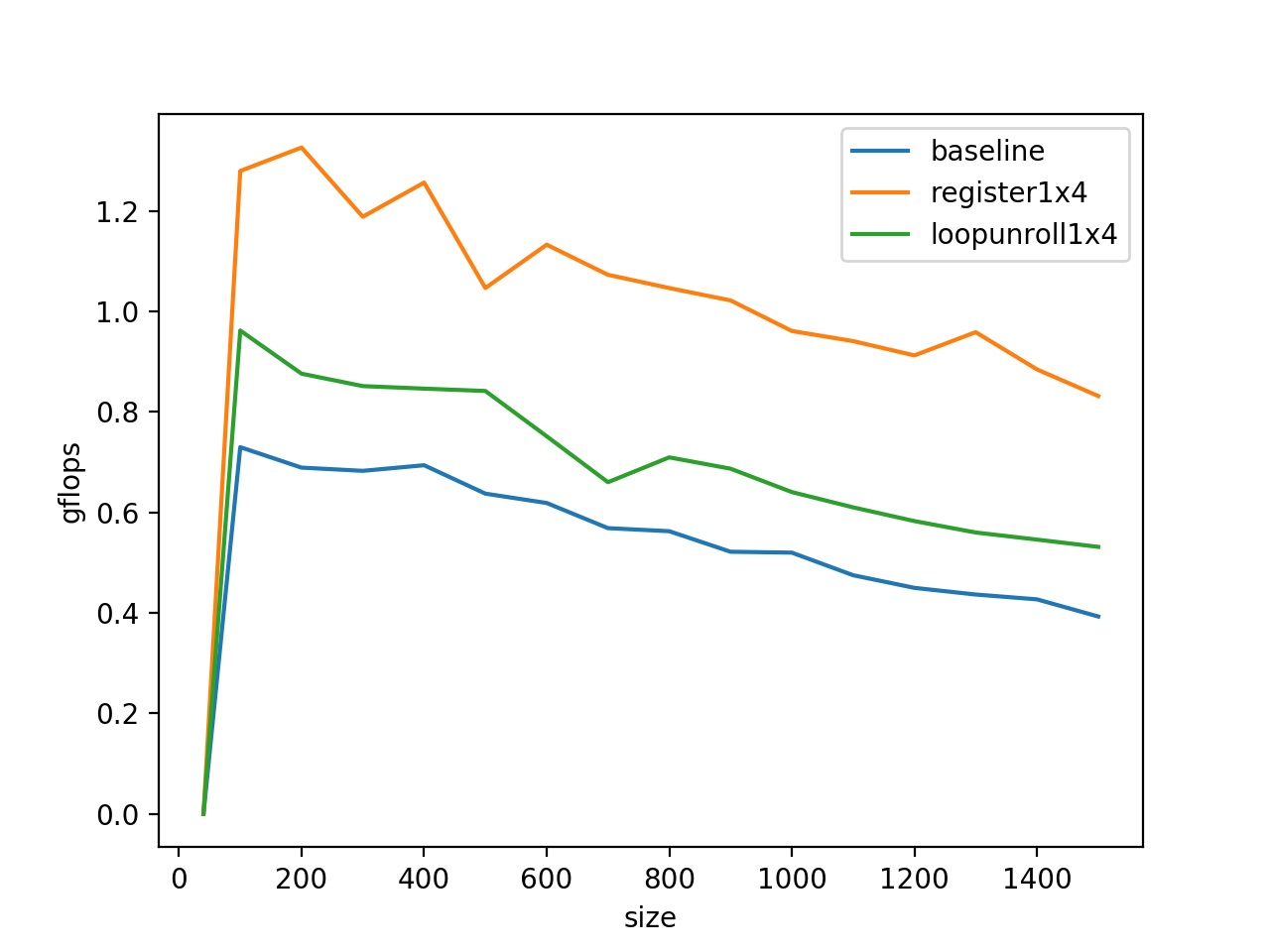### 5.SIMD指令加速

._cpufp.x86.sse.fp32.L1:
mulps %xmm0, %xmm0
mulps %xmm2, %xmm2
mulps %xmm4, %xmm4
mulps %xmm6, %xmm6
sub \$0x1, %rax
mulps %xmm8, %xmm8
mulps %xmm10, %xmm10
mulps %xmm12, %xmm12
mulps %xmm14, %xmm14
addps %xmm15, %xmm15

for ( p=0; p<k; p++ ){
a_0p_reg = A( 0, p );
a_1p_reg = A( 1, p );
a_2p_reg = A( 2, p );
a_3p_reg = A( 3, p );

b_p0_pntr = &B( p, 0 );
b_p1_pntr = &B( p, 1 );
b_p2_pntr = &B( p, 2 );
b_p3_pntr = &B( p, 3 );

/* First row */
c_00_reg += a_0p_reg * *b_p0_pntr;
c_01_reg += a_0p_reg * *b_p1_pntr;
c_02_reg += a_0p_reg * *b_p2_pntr;
c_03_reg += a_0p_reg * *b_p3_pntr;

/* Second row */
c_10_reg += a_1p_reg * *b_p0_pntr;
c_11_reg += a_1p_reg * *b_p1_pntr;
c_12_reg += a_1p_reg * *b_p2_pntr;
c_13_reg += a_1p_reg * *b_p3_pntr;

/* Third row */
c_20_reg += a_2p_reg * *b_p0_pntr;
c_21_reg += a_2p_reg * *b_p1_pntr;
c_22_reg += a_2p_reg * *b_p2_pntr;
c_23_reg += a_2p_reg * *b_p3_pntr;

/* Four row */
c_30_reg += a_3p_reg * *b_p0_pntr++;
c_31_reg += a_3p_reg * *b_p1_pntr++;
c_32_reg += a_3p_reg * *b_p2_pntr++;
c_33_reg += a_3p_reg * *b_p3_pntr++;
}b_p0_vreg.v = _mm_loaddup_pd( (double *) b_p0_pntr++ );   /* load and duplicate */
b_p1_vreg.v = _mm_loaddup_pd( (double *) b_p1_pntr++ );   /* load and duplicate */
b_p2_vreg.v = _mm_loaddup_pd( (double *) b_p2_pntr++ );   /* load and duplicate */
b_p3_vreg.v = _mm_loaddup_pd( (double *) b_p3_pntr++ );   /* load and duplicate */

/* First row and second rows */
c_00_c_10_vreg.v += a_0p_a_1p_vreg.v * b_p0_vreg.v;
c_01_c_11_vreg.v += a_0p_a_1p_vreg.v * b_p1_vreg.v;
c_02_c_12_vreg.v += a_0p_a_1p_vreg.v * b_p2_vreg.v;
c_03_c_13_vreg.v += a_0p_a_1p_vreg.v * b_p3_vreg.v;

$$C_{00} = \sum_{k=0}^{3}{A_{0k} * B_{k0}} \ C_{10} = \sum_{k=0}^{3}{A_{1k} * B_{k0}}$$### 6. 矩阵分块乘法

for ( p=0; p<k; p+=kc ){
pb = min( k-p, kc );
for ( i=0; i<m; i+=mc ){
ib = min( m-i, mc );
InnerKernel( ib, n, pb, &A( i,p ), lda, &B(p, 0 ), ldb, &C( i,0 ), ldc );
}
}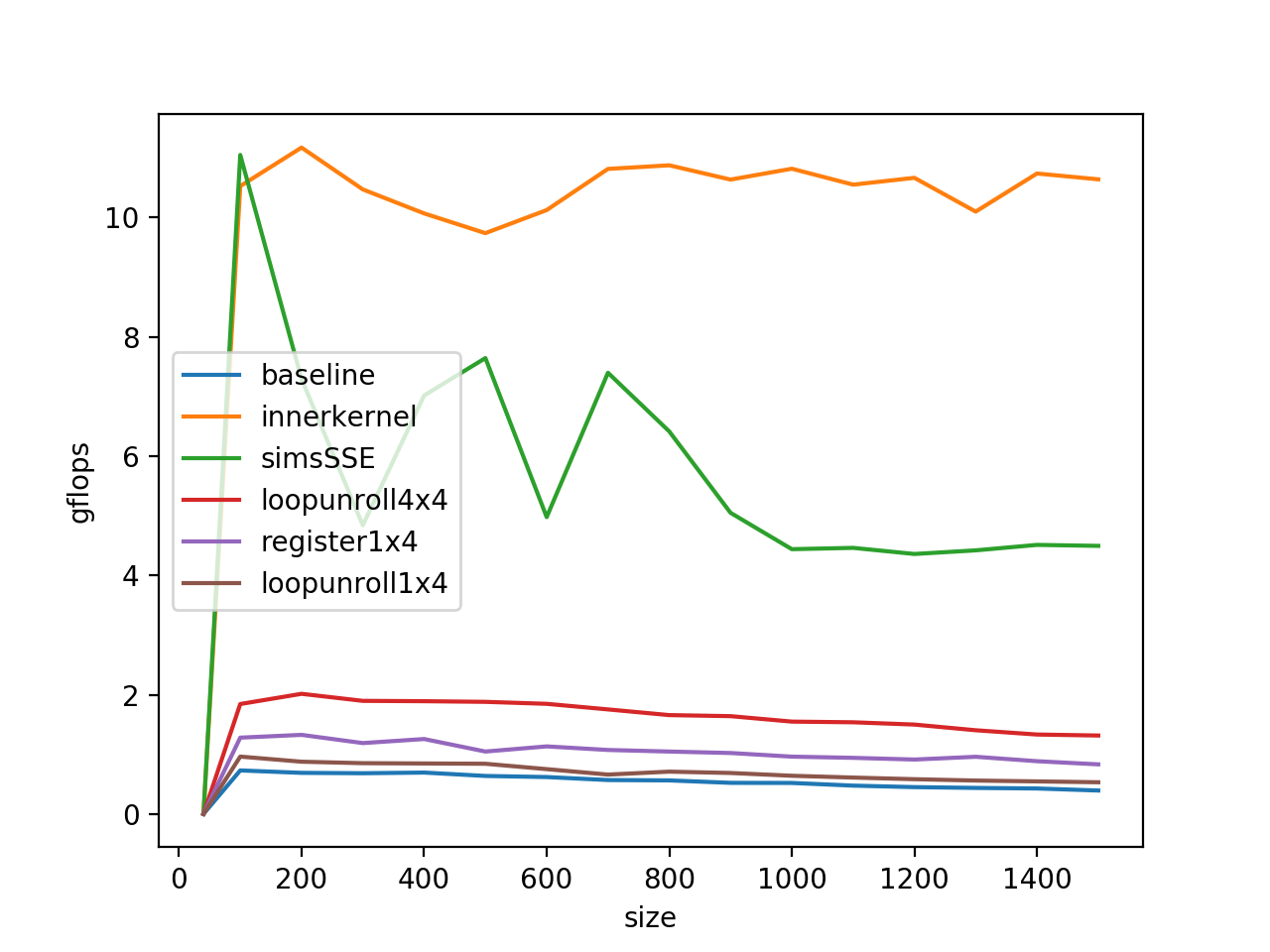### 7. DataPack